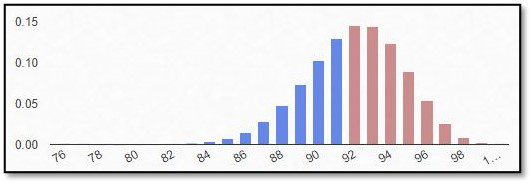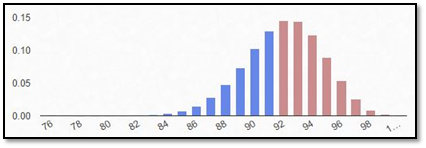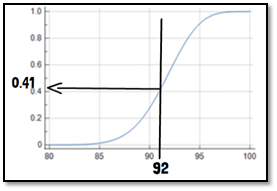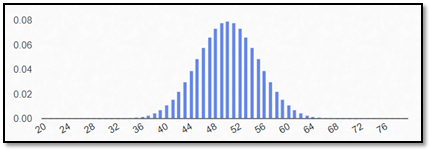# What is the binominal distribution?The binomial distribution describes, how large the probability is, that a particular event will occur in a certain number of observations. The outcome is hereby always given in only two possibilities: success and failure. Assume you want to conduct a trial where you are investigating whether a new surgery method leads to vision without additional help such as glasses. The distribution of such data is following a binomial distribution showing discrete probabilities. When including 100 patients in our trial, and assuming an expected probability of success of about 92%, the distribution of probabilities of success would look like the following.The distribution shows its peak in 92 patients with a success probability of 15%. This is the probability that exactly 92 patients finish the trial successfully. The probability of getting at least 92 successes in this trial (red bars), can be summarized from the cumulative probability function. After reading the according value from the y-axis it is subtracted from 1, resulting in a 59% probability of being able to show at least 92 successes from the 100 patients included.The binomial distribution can be approximated by the normal distribution for moderate values of the probability of success and if the number of observations is high. It can however not be used, if the number of observations or patients and the success probability is low. A rule of thumb is: if both, the number of patients times the success probability, and the number of patients times the probability of failure are larger than 10, the approximation can be used.What cannot be investigated when working with binomial distributions is the magnitude of the outcome. The differentiation is only between success and failure. In case of success, it cannot be assessed, to what degree the visual acuity, for example, was improved. This is a disadvantage that limits your outcome conclusions but also makes it easier to handle the data and simplifies the statistical analysis.

If you are interested in a visual presentation of this topic, please feel free to follow us on our GCP Mindset YouTube channel! If you would like to know more about how we could implement statistics in your clinical trial, send us a mail to statistics@gcp-service.com.

## More To ExploreBiostatistics

### What is the binominal distribution?

If an outcome variable that can be specified in two response possibilities only, data can be analyzed as binary based on a binomial distribution, where each observation of a defined number of “experiments” represents one of the two outcomes, and the probability of success is the same for each observation.
In this part of our blog series accompanying our #video #series “5 Minutes Statistics for Clinical Trials” we explain what a #binomial #distribution reflects and what are its characteristics.